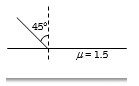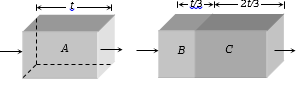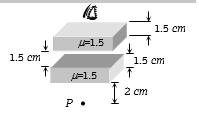A concave mirror of focal length 100 cm is used to obtain the image of the sun which subtends an angle of $30\text{'}$. The diameter of the image of the sun will be

(a)                           (b) $0.87cm$

(c)                          (d) 100 cm

Concept Questions :-

Reflection at spherical surface
High Yielding Test Series + Question Bank - NEET 2020

Difficulty Level:

A square of side 3 cm  is placed at a distance of 25 cm from a concave mirror of focal length 10 cm. The centre of the square is at the axis of the mirror and the plane is normal to the axis. The area enclosed by the image of the square is

(a)                         (b)

(c)                       (d)

Concept Questions :-

Reflection at spherical surface
High Yielding Test Series + Question Bank - NEET 2020

Difficulty Level:

A ray of light falls on the surface of a spherical glass paperweight making an angle $\alpha$ with the normal and is refracted in the medium at an angle $\beta$ . The angle of deviation of the emergent ray from the direction of the incident ray

(a) $\left(\alpha -\beta \right)$                     (b) $2\left(\alpha -\beta \right)$

(c) $\left(\alpha -\beta \right)/2$                 (d) $\left(\beta -\alpha \right)$

Concept Questions :-

Refraction at curved surface
High Yielding Test Series + Question Bank - NEET 2020

Difficulty Level:

Light enters at an angle of incidence in a transparent rod of refractive index n. For what value of the refractive index of the material of the rod the light once entered into it will not leave it through its lateral face what so ever be the value of angle of incidence

(a) $n>\sqrt{2}$                        (b) $n=1$

(c) $n=1.1$                        (d) $n=1.3$

Concept Questions :-

Total internal reflection
High Yielding Test Series + Question Bank - NEET 2020

Difficulty Level:

One face of a rectangular glass plate 6 cm thick is silvered. An object held 8 cm in front of the first face, forms an image 10 cm behind the silvered face. The refractive index of the glass is [Consider that light ray returns back in the first medium]

(a) 0.4                     (b) 0.8

(c) 1.5                     (d) 1.6

Concept Questions :-

Refraction at plane surface
High Yielding Test Series + Question Bank - NEET 2020

Difficulty Level:

One side of a glass slab is silvered as shown. A ray of light is incident on the other side at angle of incidence of ${45}^{0}$. The Refractive index of glass is given as 1.5. The deviation of the ray of light from its initial path when it comes out of the slab is:(a)   90o

(b)   180o

(c)   120o

(d)   45o

Concept Questions :-

Refraction at plane surface
High Yielding Test Series + Question Bank - NEET 2020

Difficulty Level:

Two transparent slabs have the same thickness as shown. One is made of material A of refractive index 1.5. The other is made of two materials B and C with thickness in the ratio 1:2. The refractive index of C is 1.6. If a monochromatic parallel beam passing through the slabs has the same number of waves inside both, the refractive index of B is(a)   1.1                                      (b)   1.2

(c)   1.3                                      (d)   1.4

Concept Questions :-

Refraction at plane surface
High Yielding Test Series + Question Bank - NEET 2020

Difficulty Level:

The image of point P when viewed from the top of the slabs will be(a)   2.0 cm above P

(b)   1.5 cm above P

(c)   2.0 cm below P

(d)   1 cm above P

Concept Questions :-

Refraction at plane surface
High Yielding Test Series + Question Bank - NEET 2020

Difficulty Level:

Two point light sources are 24 cm apart. Where should a convex lens of focal length 9 cm be put in between them from one source so that the images of both the sources are formed at the same place?

(a)   6 cm                                   (b)   9 cm

(c)   12 cm                                (d)   15 cm

Concept Questions :-

Lenses
High Yielding Test Series + Question Bank - NEET 2020

Difficulty Level:

(a)   2R                                      (b)   R

(c)   $\frac{3}{2}R$                                 (d)  ${R}^{2}$

Concept Questions :-

Lensmakers' formula